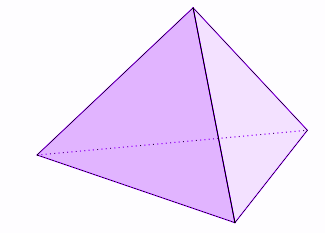QUESTION

# The faces of a triangular pyramid consist of which of the following:(a) Two triangles and three rectangles(b) Two rectangles and three triangles(c) One square and three rectangles(d) Four triangles

Hint: First, start by defining what a triangular pyramid actually is. Draw its diagram and visualise its shape. Count the number of faces and their respective shapes to arrive at the final answer.

In this question, we need to find what shape of faces does a triangular pyramid consist of and how many.
Let us first define what a triangular pyramid actually is.
In geometry, a tetrahedron, also known as a triangular pyramid, is a polyhedron composed of four faces, six straight edges, and four vertex corners. The tetrahedron is the simplest of all the ordinary convex polyhedra and the only one that has fewer than 5 faces.
The triangular pyramid has a triangle base.
And other faces attached with apex are triangles.
With every side of base, one triangle face is being created and gets attached to apex.
So, there will be 3 triangles except the base which is also triangular.
Hence, faces of a triangle pyramid consist of four triangles.
The following diagram shows a triangular pyramid:We can clearly see the four triangular faces in the above figure.
Hence, option (d) is the correct answer.

Note: In this question, it is important to visualise and understand the shape of a triangular pyramid. Also, note that the base can be any shape or size of triangle but usually it is an equilateral triangle and all the sides are the same. This means the three sides of the pyramid are the same size as each other and the pyramid looks the same if you rotate it.